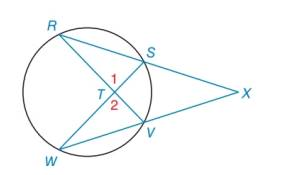Chapter 6.2, Problem 32EElementary Geometry For College St...

7th Edition
Alexander + 2 others
ISBN: 9781337614085

Solutions

Chapter
SectionElementary Geometry For College St...

7th Edition
Alexander + 2 others
ISBN: 9781337614085
Textbook Problem

In the figure shown, Δ R S T ∼ Δ W V T by the reason A A . Name two pairs of congruent angles in these similar triangles.Exercises 32, 33

To determine

To name:

The two pairs of congruent angles in the given similar triangles ΔRSTΔWVT.

Explanation

Calculation:

Given, ΔRSTΔWVT by the reason of AA similarity.

Consider the figure,

By the vertical angle theorem,

1=2.

Therefore, from the figure, SV

Still sussing out bartleby?

Check out a sample textbook solution.

See a sample solution

The Solution to Your Study Problems

Bartleby provides explanations to thousands of textbook problems written by our experts, many with advanced degrees!

Get Started

What are the two requirements for a random sample?

Statistics for The Behavioral Sciences (MindTap Course List)

Simplify the expressions in Exercises 97106. 21/32122/321/3

Finite Mathematics and Applied Calculus (MindTap Course List)

Express 58 as a decimal.

Elementary Technical Mathematics

Define induction and deduction and explain the role of each in the scientific method.

Research Methods for the Behavioral Sciences (MindTap Course List)